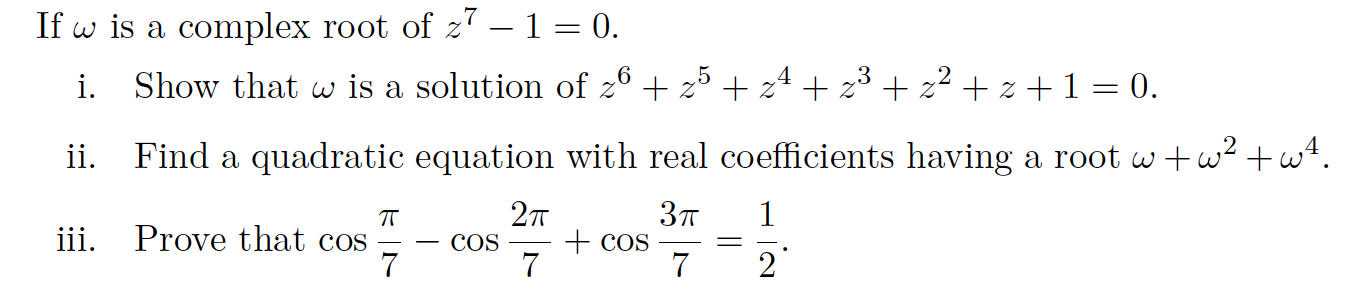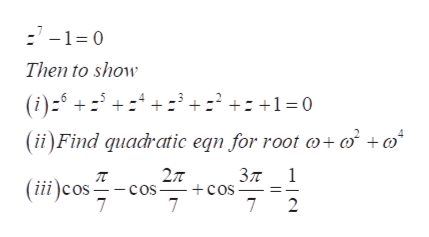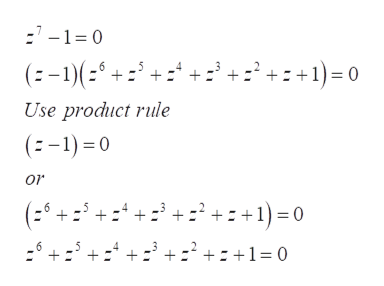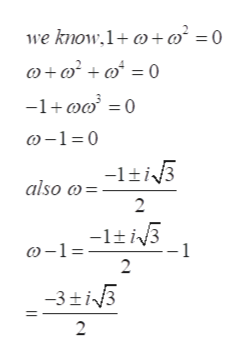# If w is a complex root of z7 - 1 = 0..5z3Show that w is a solution of 2 zzzz 1 = 0.i.Find a quadratic equation with real coefficients having a root wwwii.2тЗп1TTiii. Prove that cos7COS7COS72

Question
29 viewshelp_outlineImage TranscriptioncloseIf w is a complex root of z7 - 1 = 0. .5 z 3 Show that w is a solution of 2 z z z z 1 = 0. i. Find a quadratic equation with real coefficients having a root www ii. 2т Зп 1 TT iii. Prove that cos 7 COS 7 COS 7 2 fullscreen
check_circle

Step 1

Refer to the we need to find the show the provided equations,help_outlineImage Transcriptionclose7-1 0 Then to show (i)- 1 0 (ii)Find quacratic eqn for root o+ o + 2T - Cos 7 Зл - cos 7 1 (it)cos 7 2 fullscreen
Step 2

To solve question 1 as,help_outlineImage Transcriptionclose:'-1= 0 +:' +:+1)= 0 (:-1)(:* +. Use product rule (:-1) = 0 or :+ +:' + :' +: +1) = 0 (:' 6. +:+:+1= 0 fullscreen
Step 3

Now to solve part2 ...help_outlineImage Transcriptionclosewe know,1+ @+m² = 0 @+ w² + @* = 0 -1+@w° =0 ) -1=0 -1+i3 also @= 2 -1±i3 0-1= -1 2 -3±i3 fullscreen

### Want to see the full answer?

See Solution

#### Want to see this answer and more?

Solutions are written by subject experts who are available 24/7. Questions are typically answered within 1 hour.*

See Solution
*Response times may vary by subject and question.
Tagged in

### Math